# A Surprising Connection

At first glance, the Golden Ratio looks like it has very little to do with the Fibonacci numbers. But let’s take another close look at our Fibonacci number friends.In particular, let’s ask ourselves what happens if we divide each Fibonacci number by the one before it.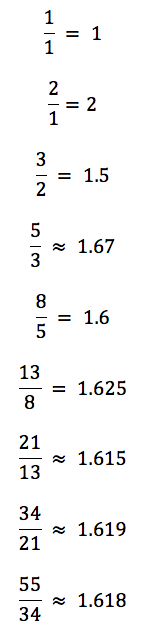You recognize 1.618? (If not, go look back at our definition of the Golden Ratio!) It certainly looks like these Fibonacci ratios are converging on the Golden Ratio. If we examine the sizes of these numbers, we might be thinking “too small… too big… kinda too small… rather too big… a bit too small… a little too big… a smidgen too small… a hair too big… almost spot-on…” If we plot these numbers on the number line we can see the convergence even more clearly: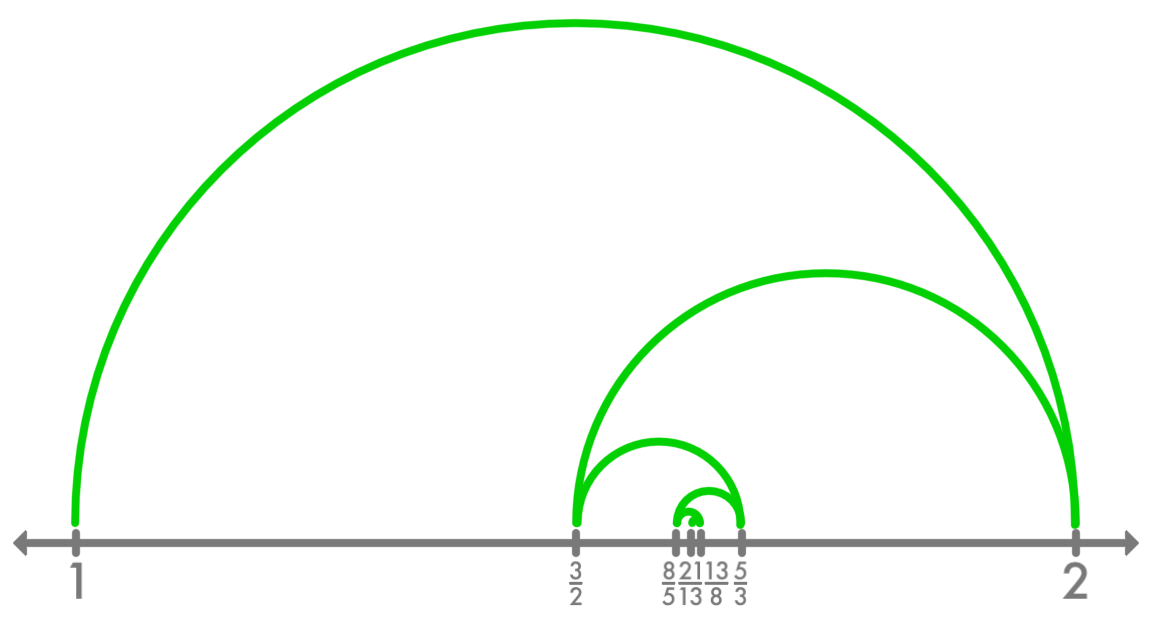As it turns out, our guess is correct: our Fibonacci ratios converge on the Golden Ratio. It’s a surprising and beautiful result. To get a better idea of why it’s true, however, we’ll need to take a detour into a problem that seems completely different.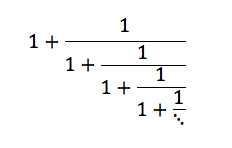Let me ask you this: What is this fraction equal to?

It’s quite an interesting puzzle. We can’t just pull out a calculator and evaluate it that way, because of the “…” that makes the fraction go on forever. Let’s experiment with this a little and try some smaller samples of this fraction. (Experimenting is very important in math – that’s how you discover things.)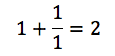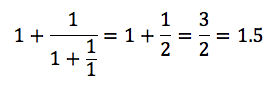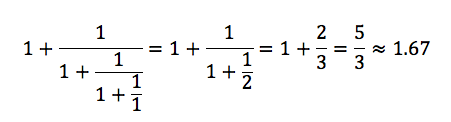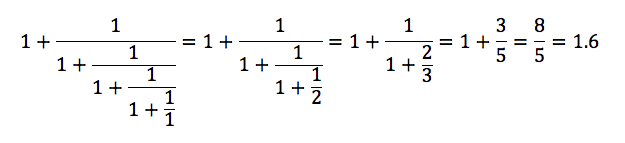Wow.

There a couple of very interesting things going on here. For one thing, those decimals seem to follow the same convergence pattern we saw a moment ago. Second, look where the decimals are coming from – the Fibonacci numbers are appearing out of this repeating fraction! (See if you can figure out why!)

What this means is that our funny infinite fraction is the same as what happens if we keep calculating Fibonacci ratios on forever. In other words, if we can prove that our infinite fraction equals the Golden Ratio, we’ll have proved that our Fibonacci ratios from the beginning of this page really do converge on the Golden Ratio.

So is it really the Golden Ratio? Can we prove it?

We can, and to do that we’ll return to the infinite fraction.We want to know what this fraction equals, so let’s call it something. If we wanted to be algebraic about it, we might use the letter x, which is generally used for unknowns. But just because we can, let’s use a question mark.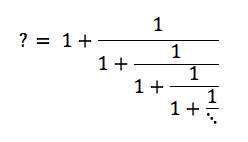Well, that’s nice, except we still have this pesky “…” to deal with. How do we work with that?

Let’s look at the fraction this way: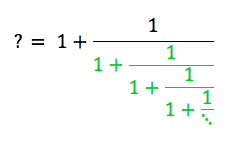What does that green part equal? It’s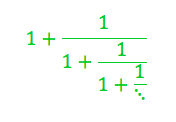It’s what we want to find! It’s the question mark! So we can rewritelike so: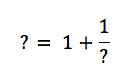Infinity can be very convenient that way. The “…” was getting in our way, so we turned the problem into the solution. (Think that was cool? Check out the page on infinity!)

Okay, so now if we can solve that equation to get the value of the question mark, we’ll have our answer. (After all, the ? represents our continued fraction ­– that’s how we defined it.) For those of you who know some algebra, try to solve the equation for the question mark.

Meanwhile, I’ll do a bit of mathemagic to give you an inkling of why we get the answer we get.

We’ll start by rewriting the number 1 in the equation as $\frac{?}{?}$ . Remember – anything divided by itself is 1. Even with carrots.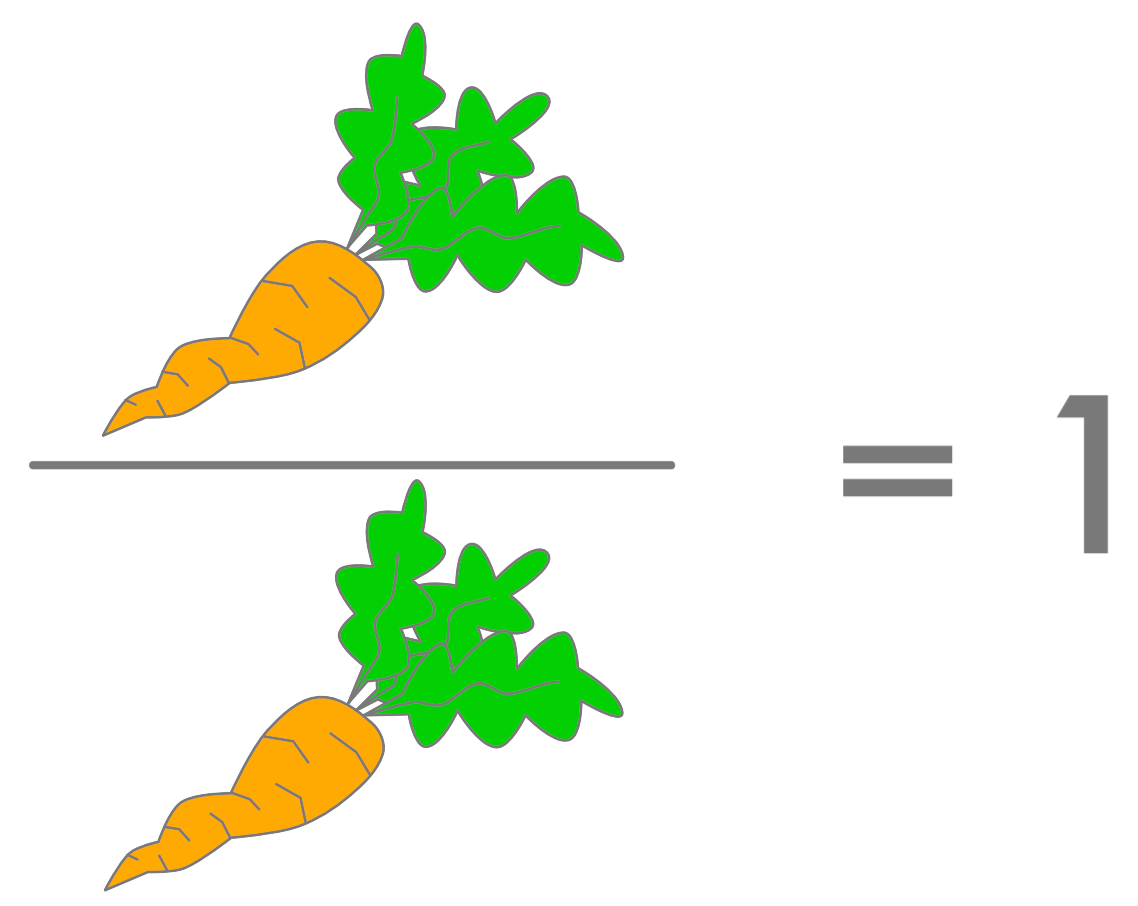So now our equation becomes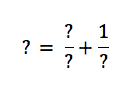And now both of those fractions have a ? downstairs, so we can add them: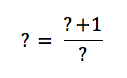Now, finally, let’s rewrite the left-hand ? as $\frac{?}{1}$. We can do that because anything divided by 1 is itself.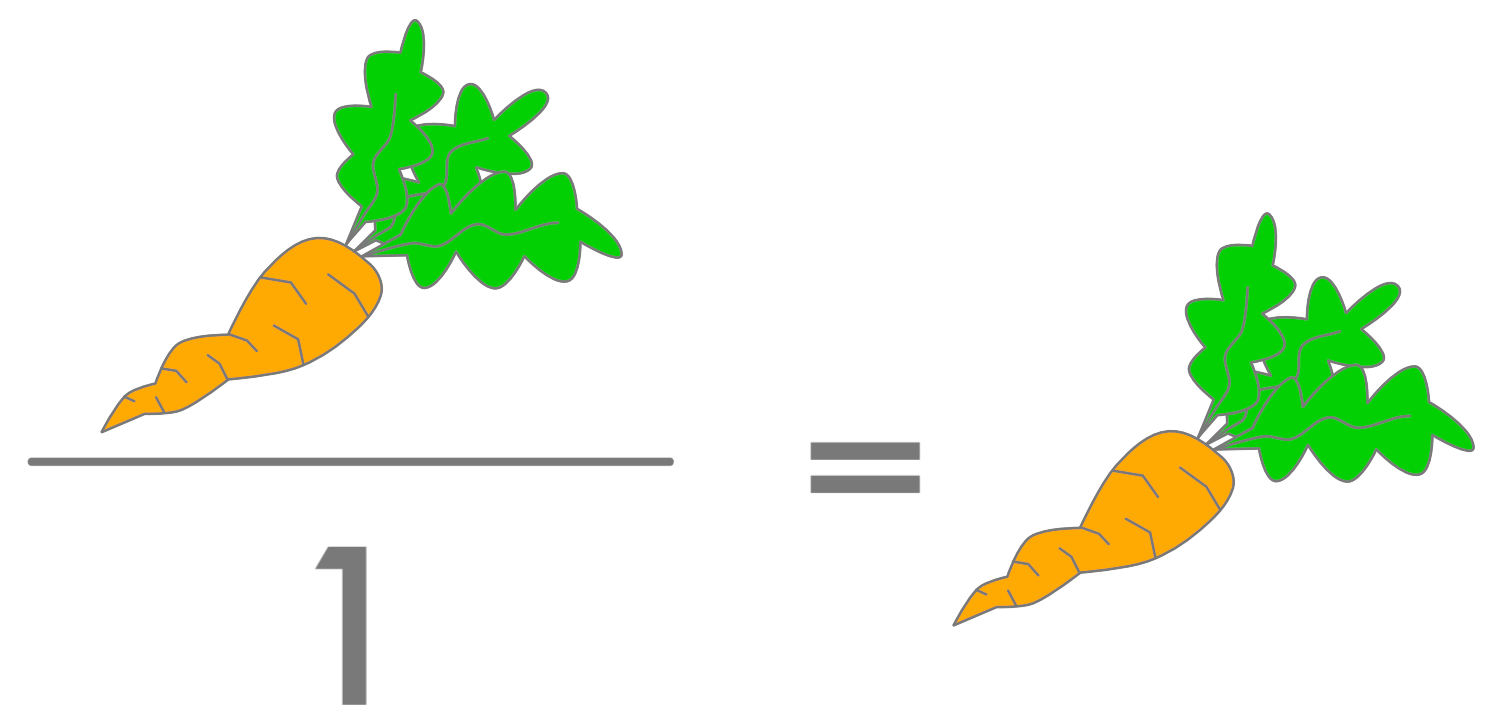And if we color the result:Green is to red as red is to blue. If that doesn’t look familiar, go look back at our original definition of the Golden Ratio!

And there we have it. Our question mark is the Golden Ratio. And so our infinite fraction is the Golden Ratio, and so the Fibonacci ratios converge on the Golden Ratio. It’s a surprising and beautiful connection. It’s also a reminder that pieces of math that at first look completely different can actually turn out to be related – if you’d like another example, try these two links!

Sierpinski

Pascal’s Triangle